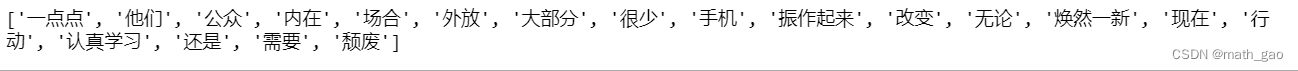﻿ Python sklearn CountVectorizer使用详解_python_脚本之家
python# Python sklearn CountVectorizer使用详解

## 数据预处理

```import jieba
import re
from sklearn.feature_extraction.text import CountVectorizer
#原始数据
text = ['很少在公众场合手机外放',
'大部分人都还是很认真去学习的',
'他们会用行动来',
'无论你现在有多颓废，振作起来',
'只需要一点点地改变',
'你的外在和内在都能焕然一新']
#提取中文
text = [' '.join(re.findall('[\u4e00-\u9fa5]+',tt,re.S)) for tt in text]
#分词
text = [' '.join(jieba.lcut(tt)) for tt in text]
text```## 构建模型

### 训练模型

```#构建模型
vectorizer = CountVectorizer()
#训练模型
X = vectorizer.fit_transform(text)```

### 所有词汇：model.get_feature_names()

```#所有文档汇集后生成的词汇
feature_names = vectorizer.get_feature_names()
print(feature_names)```### 计数矩阵：X.toarray()

```#每个文档相对词汇量出现次数形成的矩阵
matrix = X.toarray()
print(matrix)``````#计数矩阵转化为DataFrame
df = pd.DataFrame(matrix, columns=feature_names)
df```### 词汇索引：model.vocabulary_

`print(vectorizer.vocabulary_)`